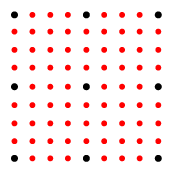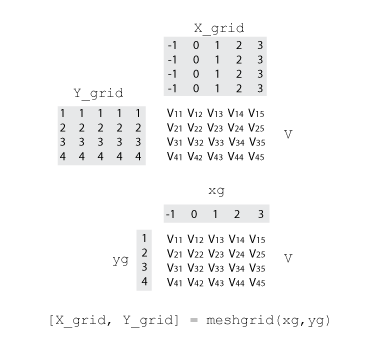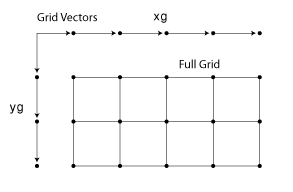# interp2

meshgrid 格式的二维网格数据的插值

## 语法

``Vq = interp2(X,Y,V,Xq,Yq)``
``Vq = interp2(V,Xq,Yq)``
``Vq = interp2(V)``
``Vq = interp2(V,k)``
``Vq = interp2(___,method)``
``Vq = interp2(___,method,extrapval)``

## 说明

``Vq = interp2(X,Y,V,Xq,Yq)` 使用线性插值返回双变量函数在特定查询点的插入值。结果始终穿过函数的原始采样。`X` 和 `Y` 包含样本点的坐标。`V` 包含各样本点处的对应函数值。`Xq` 和 `Yq` 包含查询点的坐标。`
``Vq = interp2(V,Xq,Yq)` 假定一个默认的样本点网格。默认网格点覆盖矩形区域 `X=1:n` 和 `Y=1:m`，其中 `[m,n] = size(V)`。如果您希望节省内存且不在意点之间的绝对距离，则可使用此语法。`
``Vq = interp2(V)` 将每个维度上样本值之间的间隔分割一次，形成优化网格，并在这些网格上返回插入值。`

``Vq = interp2(V,k)` 将每个维度上样本值之间的间隔反复分割 `k` 次，形成优化网格，并在这些网格上返回插入值。这将在样本值之间生成 `2^k-1` 个插值点。`

``Vq = interp2(___,method)` 指定备选插值方法：`'linear'`、`'nearest'`、`'cubic'`、`'makima'` 或 `'spline'`。默认方法为 `'linear'`。`

````Vq = interp2(___,method,extrapval)` 还指定标量值 `extrapval`，此参数会为处于样本点域范围外的所有查询点赋予该标量值。 如果您为样本点域范围外的查询省略 `extrapval` 参数，则基于 `method` 参数，`interp2` 返回下列值之一： 对于 `'spline'` 和 `'makima'` 方法，返回外插值对于其他内插方法，返回 `NaN` 值 ```

## 示例

`peaks` 函数进行粗略采样。

```[X,Y] = meshgrid(-3:3); V = peaks(X,Y);```

```figure surf(X,Y,V) title('Original Sampling');````[Xq,Yq] = meshgrid(-3:0.25:3);`

`Vq = interp2(X,Y,V,Xq,Yq);`

```figure surf(Xq,Yq,Vq); title('Linear Interpolation Using Finer Grid');``````[X,Y] = meshgrid(-3:3); V = peaks(7);```

```figure surf(X,Y,V) title('Original Sampling');````[Xq,Yq] = meshgrid(-3:0.25:3);`

`Vq = interp2(X,Y,V,Xq,Yq,'cubic');`

```figure surf(Xq,Yq,Vq); title('Cubic Interpolation Over Finer Grid');``````load flujet.mat colormap gray```

`V = single(X(200:300,1:25));`

```imagesc(V); axis off title('Original Image')````Vq = interp2(V,5);`

```imagesc(Vq); axis off title('Linear Interpolation')````[-2, 2]` 的范围内从两个维度对函数进行粗略采样。

```[X,Y] = meshgrid(-2:0.75:2); R = sqrt(X.^2 + Y.^2)+ eps; V = sin(R)./(R);```

```figure surf(X,Y,V) xlim([-4 4]) ylim([-4 4]) title('Original Sampling')````X``Y` 域范围以外延伸，创建查询网格。

`[Xq,Yq] = meshgrid(-3:0.2:3);`

`X``Y` 域内进行三次插值，并对域外的所有查询赋零值。

`Vq = interp2(X,Y,V,Xq,Yq,'cubic',0);`

```figure surf(Xq,Yq,Vq) title('Cubic Interpolation with Vq=0 Outside Domain of X and Y');```## 输入参数

• 如果 `X``Y` 是表示完整网格（`meshgrid` 格式）的矩阵，则 `V` 的大小必须与 `X``Y` 相同。

• 如果 `X``Y` 是网格向量，则 `V` 必须是一个包含 `length(Y)` 行、`length(X)` 列的矩阵。

• 如果 `Xq``Yq` 是标量，则为单个查询点的坐标。

• 如果 `Xq``Yq` 是方向不同的向量，则 `Xq``Yq` 将被视作网格向量。

• 如果 `Xq``Yq` 是大小和方向均相同的向量，则 `Xq``Yq` 将被视作二维空间中的散点

• 如果 `Xq``Yq` 是矩阵，则表示由多个查询点构成的一个完整网格（`meshgrid` 格式）或是多个散点。

• 如果 `Xq``Yq` 是 N 维数组，则表示二维空间中的散点。

`interp2(V,1)``interp2(V)` 相同。`'linear'`对查询点插值基于各维中邻点网格点处数值的线性插值。这是默认插值方法。C0
• 每个维度需要至少两个网格点

• `'nearest'` 需要更多内存

`'nearest'`在查询点插入的值是距样本网格点最近的值。 不连续
• 每个维度需要两个网格点。

• 内存要求最低，计算速度最快

`'cubic'`对查询点的插值基于各维中邻点网格点处数值的三次插值。插值基于三次卷积。C1
• 每个维度中的网格必须有均匀间距，但并非所有维度的网格间距都必须相同

• 每个维度需要至少四个点

• `'linear'` 需要更多内存和计算时间

`'makima'`修正 Akima 三次 Hermite 插值。对查询点的插值基于次数最大为 3 的多项式的分段函数，使用各维中相邻网格点的值进行计算。为防过冲，已修正 Akima 公式。C1
• 每一维需要至少 2 个点。

• 产生的波动比 `'spline'`

• 计算时间通常少于 `'spline'`，但内存要求类似

`'spline'`对查询点的插值基于各维中邻点网格点处数值的三次插值。插值基于使用非结终止条件的三次样条。C2
• 每个维度需要四个点

• `'cubic'` 需要更多内存和计算时间

`X``Y` 域范围外的函数值，指定为实数或复数标量。`interp2``X``Y` 域范围外的所有点返回此常量值。

## 输出参数

`interp2(X,Y,V,Xq,Yq)`
`interp2(V,Xq,Yq)`

`Xq``Yq` 是标量标量`Xq``Yq` 作为标量传递时，`size(Vq) = [1 1]`

`size(Yq) = [100 1]`
`size(Vq) = [100 1]`

`size(Yq) = [50 1]`
`size(Vq) = [50 100]`

`size(Yq) = [50 25]`
`size(Vq) = [50 25]`
`method` 以及包含 `extrapval``interp2(V,k)`

`2^k * (size(V,1)-1)+1`

`2^k * (size(V,2)-1)+1` 的矩阵

`k = 2`
`size(Vq) = [37 77]`

## 详细信息

### 完整网格（meshgrid 格式）

`interp2` 而言，完整网格是一对矩阵，其元素表示矩形区域内的网格点。一个矩阵包含 x 坐标，另一个矩阵包含 y 坐标。x 矩阵中的值沿行方向严格单调递增。列方向上表现为常量。y 矩阵中的值沿列方向严格单调递增。行方向上表现为常量。使用 `meshgrid` 函数创建可传递至 `interp2` 的完整网格。

`[X,Y] = meshgrid(-1:3,(1:4))`
```X = -1 0 1 2 3 -1 0 1 2 3 -1 0 1 2 3 -1 0 1 2 3 Y = 1 1 1 1 1 2 2 2 2 2 3 3 3 3 3 4 4 4 4 4```### 网格向量

`interp2` 而言，网格向量由一对定义网格 x 和 y 坐标的向量组成。行向量定义 x 坐标，列向量定义 y 坐标。```x = -1:3; y = (1:4)';```

### 散点

`interp2` 而言，散点由一对定义二维空间散点集合的数组组成。一个数组包含 x 坐标，另一个数组包含 y 坐标。

```x = [2 5; 4 10]; y = [7 3; 1 9];```